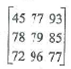# Data Structures

Computing

## Quiz 1 : Software EngineeringStudy FlashcardsLooking for Programming Homework Help?

## Quiz 1 :Software Engineering

Question TypeName three kinds of programming errors and give examples of each. When during program development is each likely to be detected?
Free
Essay

The three kinds of programming errors:
• Syntax errors
• Run-time errors
• Logical errors
• Syntax errors:
These errors occur in the grammatical rules such as missing semicolons, misspelled words and declarations part is absent.
Example:
int number syntax error; because semicolon is missing in the end
cin number;
• Run-time errors:
A Runtime error occurs when execution of a program. The runtime errors are divisible by zero, illegal memory access, and try to determine the square root of a negative number.
Example:
int p = 40, q = 20, r = 20;
int answer = p / (q - r); Run-time error; because it's divisible by zero
int result = p / (q + r);
• Logical error:
In logical error, program execution is correct however the corresponding program does not generate the exact output.
Example:
Totalmonths = 13;
result = Total / Totalmonths;
In the above example, program executed successfully, but it will generate the wrong output; because the "Totalmonths" is 12 but in the above example Totalmonths = 13.

Tags
Choose question tagWhat is pseudocode?
Free
Essay

Pseudo code:
The Pseudo code is a combination of normal language with symbols that can be used as procedure for the solving the problem.
It is generally written in common language to understand the terms and does not require any syntax for defining the problem which makes it easy for better understanding of problem and could be easy in implementing in one or more high level programming languages.
Example:
The following pseudocode is used to find the maximum of two numbers:
if a is greater than b then
print a is maximum
else
print b is maximum

Tags
Choose question tagWhy is the following correct program segment not a good solution to Problem 1? (Consider its efficiency.) ? / * Search the entries of the n × n matrix mat in rowwise order for an entry equal to item * / bool found = false; for (int row = 0; row n; row++) for (int col = 0; col n; col++) if (mat[row][col] == item) found = true; if (found) cout "item found\n"; else cout "item not found\n";
Free
Essay

Analysis:
When analyzing the given program segment, it is used to check the whether the given search item is found in the given matrix or not. If the search item is found, it returns true. Otherwise returns false.
Reason:
In the problem 1, the variable "found" is not declared and initialized which means "bool found = false" is missing in the given program.
Another reason is that cannot use the goto statement in given program which is not structured.
Explanation:
• The given program is used to check whether the search item is found in the given matrix or not.
• The given program is not efficient to problem 1. Because in this program, the matrix is searched completely and find out the given searched value is present in the matrix or not using "found" variable.
• But in the problem 1, variable "found" is not declared and initialized. So that program cannot return the given search item is found or not.
• So given program is not efficient to problem "1". After inserting the "bool found = false", it will return the search item.

Tags
Choose question tagWhat are the three characteristics of object-oriented programming?
Essay
Tags
Choose question tagWhat are some of the ways in which student programs differ from real-world software?
Essay
Tags
Choose question tagName and describe the three control structures used in developing structured algorithms.
Essay
Tags
Choose question tagName and describe two design methodologies.
Essay
Tags
Choose question tagDefine an algorithm, naming and describing the properties it must possess.
Essay
Tags
Choose question tagWhat are some situations in which maintenance may be required?
Essay
Tags
Choose question tagAlthough the following program segment is structured, it is not a correct solution to Problem 1: / * Search the entries of the n × n matrix mat in rowwise order for an entry equal to item * / bool found;for (int row = 0; row n; row++) for (int col = 0; col n; col++) if (mat[row][col] == item) found = true; else found = false;if (found) cout "item found\n";else cout "item not found\n"; a. Write a program that incorporates this program segment and then perform black-box testing of it, beginning with the matrix mat =and item = 77 as the first set of test data. Produce enough output to show that your testing has turned up an error. b. Use program tracing to determine what is wrong with this program segment.
Essay
Tags
Choose question tagWhat are structured data types? Give an example from C++.
Essay
Tags
Choose question tagName and describe the five phases of software development.
Essay
Tags
Choose question tagThe following function performs a linear search of a list l of length ll for the item it, returning 0 or 1 depending on whether it is not found. Many principles of good programming are violated. Describe some of these and rewrite the function in an acceptable format. int LS(int l[], int ll, int it) / * Search l for it * / {int i=0, f=0; A:if (l[i]==it)goto B; if (i==ll) gotoC; / *ADD 1 TO i* / i++;goto A;B:f=1;C:return f;}
Essay
Tags
Choose question tagFind some other examples of "software horror stories" and write brief reports for each, describing the error and what harm or adversity resulted from it.
Essay
Tags
Choose question tagWhat are some of the ways in which problems in introductory programming courses differ from real-world problems?
Essay